﻿ Enhancing the Convective Heat Transfer Coefficient Using a Single or Doubled StrainersPublications are Open
Access in this journal
Article Versions
Export Article
• Normal Style
• MLA Style
• APA Style
• Chicago Style
Original Article
Open Access Peer-reviewed

### Enhancing the Convective Heat Transfer Coefficient Using a Single or Doubled Strainers

Saeed J. Almalowi
American Journal of Mechanical Engineering. 2020, 8(2), 69-75. DOI: 10.12691/ajme-8-2-4
Received July 17, 2020; Revised August 19, 2020; Accepted August 28, 2020

### Abstract

Numerical investigation has been employed using Lattice Boltzmann Model (LBM) to investigate a temporal and a spatial characteristics of the flow past over a single and doubled strainers confined in a channel. A two dimensional nine directional (D2Q9) lattice arrangements is employed. The single and doubled strainers have been considered for Reynolds numbers Re=100. Flow and temperature field of a single and doubled strainers have been predicted. The local and the average Nusselt number have been evaluated for a single and a doubled arrangement of strainers. The results illustrate the effect of the porosity of the strainer on the local and the average Nusselt number. Consequently, a strainer enhances the heat transfer coefficient inside the A/C duct.

### 1. Introduction

Channel with screening has been widely applied in a various engineering applications such as water flow filtering or A/C filtering placed is a duct…etc. The Lattice Boltzmann method has been applied here to study a single and a doubled screening fitted inside the channel. In the reviewing literature survey, I found that LBM is a tool from statistical mechanics, which is used to predict macroscopic properties of fluids such as viscosity, thermal conductivity, and diffusion coefficient from the microscopic properties of atoms and molecules. The method has been investigated by several investigators and has been increasing in popularity since its development in the early nineties. Chen and Doolen 1 accounts the history of the Lattice Boltzmann Method (LBM) and its evolution from the old Lattice Gas Automata method. They go on to discuss some of the classical flows studied with LBM such as: multiphase flows, suspended particles, and heat transfer problems. LBM has been shown to have accuracy comparable to traditional CFD methods. Since its initial application using a single relaxation time, the method has been modified in a number of ways to improve its stability. Two well-known modifications are the Multi-Relaxation Time method (MRTLBM) and the Regularized method(RLBM). MRTLBM gained some traction especially with higher Reynolds number flows. The present investigators 2 have studied Raleigh-Taylor Instabilities with MRTLBM. In multi-phase applications, such as the Raleigh-Taylor Instability, single relaxation time LBM can easily become unstable at low viscosities. MRTLBM improves the stability of this method enough to cover this problem. McCracken and Abraham 3 also work around this stability problem when studying two-phase oscillating liquid cylinders problem using the MRTLBM. There are far fewer examples of the Regularized method being used. Izham et al 4 demonstrates the Regularized LBM on a high Reynolds number lid driven cavity flow.

In the present investigation, MRTLBM and RLBM are combined to further improve the models stability. The flow this method will be tested upon, is the classical flow past a square cylinder. This flow was chosen because it was an easy to program geometry which produces complicated turbulent structures at high Reynolds numbers. The flow past a cylinder is well studied, so our results were compared to other numerical results obtained through large eddy simulation (LES) and experiments. Once the validity of the flow past a square cylinder was established, the present investigators also studied the flow past an array of square cylinders, both inline and offset from one another.

### 2. Mathematical Model

The Lattice Boltzmann Method (LBM) has been derived from the Lattice Gas Automaton method (LGA) several years ago. LBM methodology studied by Martin et al. 5 and by Wolf-Gladrow 6. In LGA imaginary particles are placed on the lattice and are given velocities. At each time step the particles travel along the lattice to their next position based on their velocities, it is called the propagation step. Then, if multiple particles are on the same node in the lattice, certain collision rules are followed, it is called the collision step and it is in this step that the velocities are updated for the next time step. The two steps satisfy the conservation of mass and momentum. Mass is conserved by maintaining the same number of imaginary particles throughout the simulation and momentum is conserved by the rules set in the collision step. However, there are serious problems with noise due to the bulk treatment of the particles and the viscosity is hard to control due to how collisions are treated. LBM utilizes Boltzmann’s density distribution function to create a continuous model for the particles which introduces a relaxation term. Thanks to this relaxation term and the fact that LBM uses a continuous distribution function the problems with noise are smoothed out and the viscosity becomes easy to set.

Before we continue into the discussion of LBM there are a few issues that should be addressed. The lattice variables, while typically retaining the same or similar notation as their real counterparts are not the same. As outlined by Succi 7, the general rule is to obtain the lattice parameters using the discretization parameters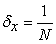and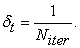Notice that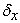is simply the reference length divided by the number of cells and that in this normalized situation the reference length is taken to be one. Similarly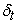is simply the reference time, again taken be one, divided by number of iteration. In effect this means thatis the spacing between two adjacent lattice nodes and thatis the time of a single time step.

A description of the lattice structure is also necessary before continuing. In LBM research it is common to refer to the lattice being studied as DnQl, where n and l are numbers. In this shorthand, the n is the number of spatial dimensions and the l is the number of lattice directions at each node. The present study was done using D2Q9, which is depicted in Figure 1.

• Figure 1. D2Q9 arrangement for lattice inside the flow domain

The Boltzmann relation which all of LBM research is based on is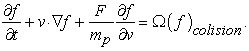(1)

In equation (1) vis the particle velocity, F is the term of body forces, and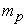is the particle mass. Note that the third term will be dropped as the present investigation contains no body forces.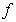is the density distribution function, which is to say, for some position and some time, the number of particles per volume with a velocity between v and v + dv This form of the equation is, unfortunately, unusable since the time rate of change ofdue to collisions on the right hand side is not easy to be determined. In the 1950s Bhatnagar et al. 8 introduced the approximation:(2)

Here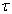is the lattice relaxation time; which is, in the single relaxation time method below, determined by viscosity and the lattice parameters. The Boltzmann equation with this relation, sometimes called the BGK model, is what most LBM research is based on. The original research into the modern LBM was done on the single relaxation time method. Martinez 9 verifies the results of the method by comparing his results for a shear flow with results obtained by a spectral method. Hazi and Jimenez 10 verify a modified version of this equation for 2-D decaying turbulence in a square box against pseudo-spectral methods. Single relaxation time LBM is still popular in research due to the ease with which it is implemented. In single relaxation time LBM the propagation and collision steps are represented in the evolution equation.

In equation 3 above, the left hand side represents propagation and the right hand side represents the collision. Where the function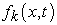is the density distribution function along the lattice branch k. x is the spatial coordinate and t is time.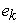is the expression for the discrete velocities along each lattice branch k.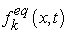is the equilibrium distribution. This is a very important term, since the appropriate selection of this function guarantees that the evolution equation satisfies the Navier Stokes equation. To select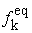we must apply a Chapman-Enskog expansion, outlined in Chapman and Cowling 11, which gives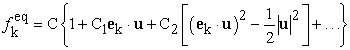(4)

whereis the fluid velocity. The higher order terms are unnecessary for an incompressible fluid; therefore only three constants (C, C1, C2) need to be selected. To select these constants these three constraints from kinetic theory are applied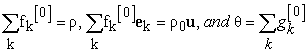(5)

which are necessary to satisfy the conservation laws. Here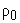is the density of the fluid and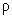is the local density. While the fluid in question is incompressibleis capable of small variation because in this methoddefines pressure as described below. After applying constrains (equation 5) to the Chapman-Enskog expansion (equation 4), it yields: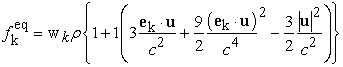(6)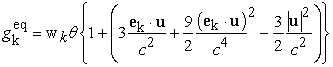The full proof that the evolution equation satisfies the Navier Stokes equation can be found in He and Lou 12; however for the sake of understanding the RLBM discussed below a brief overview must be provided. The first step is to express the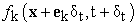terms in 2-D Taylor series expansion as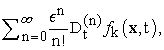where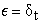is a small parameter and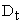is the operator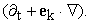Then from perturbation analysis the function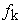can be expressed as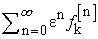and the operator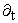as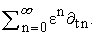Then substitute these definitions into the evolution equation and extract the terms of order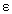zero, one and two. By applying the constraint equations above and with the definition of dimensionless pressure as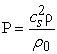the first order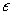equation can be shown to be the Euler equation and the second orderequation can be shown to be the full Navier Stokes equation.

Single relaxation time LBM has a great deal of stability issues and will begin to fail even at fairly low Reynolds numbers. To solve these stability issues past investigators (d’Humieres 13) developed the Multi-Relaxation Time Lattice Boltzmann Method (MRTLBM). At the start of the present study MRTLBM was employed to deal with the high Reynolds numbers in the problem. In MRTLBM the evolution equation becomes the system of equations: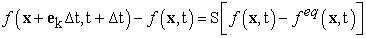(7)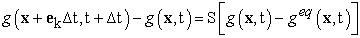Where the bold face is used to denote a vector of k elements and S is a matrix called the collision matrix. Notice that when the collision matrix is defined as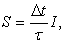where Iis the identity matrix, the equation reduces to the single relaxation time LBM evolution equation. In MRTLBM the idea is to go from the velocity space whereexists to a moment space where the function m exists. Note that the vector m is made up of elements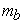where the set b has the same cardinality as the set of k. Each momentcan be found using the relation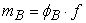(8)

This definition clearly shows that the moments are linear combinations of f. From this, basic linear theory tells us that the velocity space and the moment space must have a linear mapping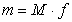(9)

Now choose the collision matrix in such a way that its eigenvectors are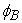the collision process will be naturally accomplished. Applying these relations to equation (7) will result in the MRTLBM evolution equation.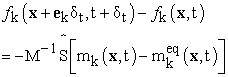(10)

Where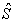is a diagonalized new collision matrix such that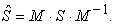For a more detailed derivation, please refer to d’Humieres and his colleagues 13, 14.

A number of years ago another method was developed from single relaxation time LBM; this method, called Regularized Lattice Boltzmann Method (RLBM), was an attempt to improve the stability of single relaxation time LBM by using a non-equilibrium distribution function. However as MRTLBM grew more popular the method got less attention. In the classical RLBM, a non-equilibrium distribution function is introduced such that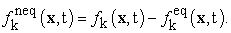(11)

Similarly a non-equilibrium stress tensor is found as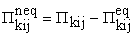(12)

Now introduce this definition for the relationship between the stress tensor and distribution function, as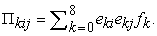(13)

Notice that this is simply the velocity moment of the distribution function. Combining these two equations gets the following relation for the non-equilibrium part of the stress tensor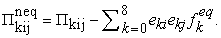(14)

Now in the interest of canceling higher order contributions to our governing equation as described in Latt and Chopard 15 the following expression if found using the Chapman-Enskog expansion.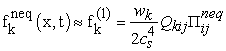(14)

Where the tensoris defined as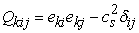and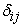is the Kronecker delta function. Using this equation the modified evolution equation of the form is obtained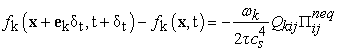(15)

Hayashi found a good permeability agreement between LBM with and without an external force on flow simulation over 3-D Nafion polymer membrane 16.

Narvaes, Ariel, et al. applied LBM to flow in porous media at low, moderate and high Re and achieved a good numerical agreement with FEM results to reproduce Forchheimer’s law at high Re and Darcy’s law at low Re 17. Grucelski and Pozorskiused LBM for flow and heat calculations and showed a consistency between the results (pressure loss vs. porosity) and the empirical laws of flow past a porous media. Additionally, confirms qualitatively that the average temperature variations for fixed and variable geometry are accurate 18.

Pan, C., et al. obtained a good agreement between LBM results on two-phase flow in porous media and the experimental hysteretic capillary pressure saturation relations 19. Edo Boek and Venturoli Maddalena applied LBM to flow in complex 3D porous media and concluded that Darcy’s law covers a wide scope of forcing values, permeability depends on the rock size sample, and permeabilities in binary mixtures depend on the forcing conditions 20. Thoms, Martin L.R., utilized LBM on stokes flow over unimodal fibrous porous media and realized for small solid volume fractions (SVF), LBM results are fairly consistent with the literature data including rough lattices and declines rapidly with higher SVF 21.

2.1. Boundary Conditions

LBM is a transient simulation method, so it follows that at any time step the model can be paused and the operator would have the solution at some real time. However, this is not necessarily true, at very short run times erroneous results can be seen. These errors are due to a mistreatment of the initial conditions. If a solution very far down in time is pursued, choice of initial conditions has no significant influence. So long as boundary conditions are correctly set up and the stability of the flow is not a problem, the flow will converge to the correct solution. However if the solution of interest is only a few time steps from the beginning of computation or, as in our case stability is already an issue, then some careful treatment will be required. Skordos 22 studies the initial condition problem and shows that accuracy at short times can be vastly improved by using an extended collision operator as outlined in his paper. While this would be an interesting area for future study, in this study a simpler method is implemented the entire computational domain is set to the value of the equilibrium function. Initially there is no flow in the computational domain the density is equal throughout; therefore thevalues are simply the weighting function at all points in the computational domain.

• Figure 2. D2Q9 arrangement for the lattice located on the bottom boundary

Accurate treatment of boundary conditions has long been a problem in LBM. For Figure 2, where a lattice is on the bottom boundary with no slip and no penetration, at each time step directions 4, 2, 7, 3, and 6 can be found using the same evolution equation as any interior node. However, lattice directions 8, 1, and 5 would be streaming from nodes which do not exist. A number of methods have been developed to treat these nodes. Early on the first order bounce-back method, as outlined in Gallivan et al. 23, grew popular due to its simplicity and ease of use. In this method any component of the particle distribution function which goes into a wall is reflected back to the opposite direction. In our example, directions 8, 1, and 5 can be obtain from the previous values of 6, 3, and 7, respectively. While this method works for simple simulations, it has a low order of accuracy which makes it a bad choice for problems where stability is an issue. The bounce-back method can be improved with what is called the second order bounce-back method as outlined by Kim 24. This method works on the same way as the simple bounce-back but the location of the bounce-back process is shifted off the boundary to a set of lattice sites placed halfway between the wall and the previous set of nodes. These two methods can be modified for moving boundaries and various other problems. However, there exists a method of dealing with the boundary conditions studied by Zou and He 25 and by Kuo and Chen 26 which is more general. The idea is to solve the system of equations formed by: the conservation of mass, the conservation of momentum (equation 5), and equations for the bounce-back of only the non-equilibrium part of the particle distribution function normal to the boundary.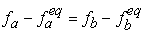where b is opposite of a. In the no slip and no penetration case shown in Figure 2 this generates the equations: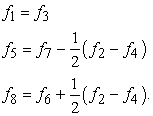(16)

This method of deriving the boundary conditions from the conservation laws is very versatile. The same style of derivation can be used to form boundary conditions for moving boundaries, penetration on the boundaries, or boundaries where pressure specified instead of velocity. This is the method used in the present investigation.

2.2. Flow Geometry

In this paper, 2D geometries are being studied. The first is a channel flow over a single strainer. The second is a channel flow two strainers placed directly behind one another. H is the channel height. The channel length, L, to height, H, aspect ratio is fixed as 10 in all geometries.

Also common to all geometries is the inlet flow profile, which is taken to be a parabolic velocity with the maximum velocity,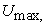defined by the Reynolds number,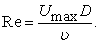No slip boundary conditions are assumed on the top and bottom boundaries for all geometries. The lattice arrangement is the D2Q9 arrangement discussed above. The arrangements of a single and a doubled strainers and the distance between centers of two strainers are shown in the Figure 3(b) and normalized by the length channel.

• Figure 3. Schematics diagram of the two studied cases (a single and doubled strainers)
2.3. Porous Media Methodology

The governing equation for the strainers has been treated as the porous media. The external forces in the equation (1) can be treated from Darcy law as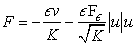(17)

The geometric function Fε and the permeability K of the porous medium are related to the porosity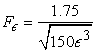(18)

The permeability is defined as: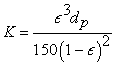(19)

The Darcy number can be written as: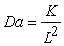where L is the characteristic length (in y-direction).

The porosity can be treated constant or various in the y-direction or x-direction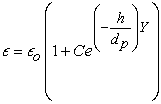(20)

The porosity diameter can be calculated based on the Darcy number as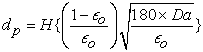(21)

From equation (20) we can find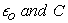based on the following conditions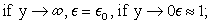In this study two cases has been studied, case (1) with constant porosity along span wise direction and case (2) when the porosity changes along span wise direction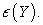The local and average Nusselt number can be calculated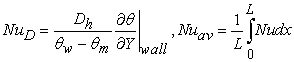(22)
2.4. Convergence and Validation Tests

The spatial and temporal tests have been illustrated for different number of lattices as shown the Figure 4a. The number of lattices selected to be Ny=120 lattices and AR=10 to be sure the results are independent on lattices. The stream wise velocity at X=0.5 plotted against those obtained by analytical solution of the velocity as shown in Figure 4b without strainer.

• Figure 4. a. Stream wise velocity plotted at X=0.5 for different number of lattices b. streamwsie velocity at X=0.5 compared by those obtained analytically

### 3. Results and Discussion

The velocity boundary conditions are shown in the schematic diagram in the Figure 3, placed to be no-slip boundary condition in the upper and lower surfaces. Inlet velocity is parabolic (U=4Uav(Y-Y2)) where Uav=1.5 and the outlet boundary condition is placed to be outflow 27. The channel is heated from the upper and lower surface (θ=1) and the inlet is placed at θ=0.5 when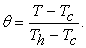The heated channel gets cold flow from the inlet as shown in the schematic diagram in the Figure 3. The effect of the screening in the temperature distribution inside the duct can be observed in the temperature contour as shown in the Figure 5. Selecting different porosity of the filtering can enhance the heat transfer coefficient h.

In this part of study, the porosity is a function of y, which means that the porosity changes in span wise direction. The comparison between the local Nussle numbers for different porosity is shown in the Figure 7. There is small variation with those obtained by porosity of 0.5 which means that the local Nusselt number at a certain condition (when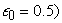will not change too much.

• Figure 5. Instantaneous temperature contour of a. single strainers and b. two strainers of Re=100 and porosity ε=0.4
• Figure 6. Dimensionless Nu number of two strainers of Re=100 of ε=0.4 and ε=0.5
• Figure 7. Dimensionless local Nu number of the two strainers of Re=100 of a constant and a changed porosity
• Figure 8. Average Nusselt number at various porosity of a single and two strainers
• Figure 9. Stream wise velocity at various porosity of a single strainer

Figure 8 shows the linear regression between the average Nusselt number and the porosity for a single and doubled strainer, it is observed that Nusselt enhances when the number of strainers increases. The effects of the porosity of the filter on the velocity field at the middle of the channel can be seen in the Figure 9. Therefore, if the porosity of the filter increases, the velocity of the air passes through the A/C duct will increase as well.

### 4. Conclusion

SRT-Lattice Boltzmann method was implemented to study a single and doubled strainers. The two studied cases proved that the porosity of the filtering fitted inside A/C duct enhanced the heat transfer coefficient as well as supported the duct structure. The local and the average Nusselt number evaluated either for constant porosity or changed porosity in y-direction. D2Q9-SRT gave stable and accurate results, but it fails for high Reynolds number. Therefore, Smagronisky model combined with LBM can improve the stability, which is undergoing now to push the simulation for high Reynolds number.

### Acknowledgements

The author would like to acknowledge the support of the Deanship of Scientific Research at Taibah University for financial and logistic support under the research group No. 60300.

### References

  S Chen and G D Doolen, “Lattice Boltzmann Method for Fluid Flows,” Annu. Rev. Fluid Mech, vol. 30 p.329-364, 1998. In article View Article  S J Almalowi, D E Oztekin and A Oztekin, “Rayleigh-Taylor Instability Studied with Multi-relaxation Lattice Boltzmann Method”, ASME Conference Proceeding IMECE2013, San Diego, CA, 2013. In article View Article  M. McCracken and J. Abraham, “Multiple-Relaxation-Time Lattice-Boltzmann Model for Multiphase Flow,” Physical Review E, vol. 71, no. 3, 2005. In article View Article  PubMed  M Izham, T Fukui, and K Morinishi, “Application of Regularized Lattice Boltzmann Method for Incompressible Flow Simulation at High Reynolds Number and Flow with Curved Boundary,” Journal of Fluid Science and Technology, vol. 6, no. 6, 2011. In article View Article  O Martin, A Odlyzko, and S Wolfram, “Algebraic Properties of Cellular Automata”, Communications in Mathematical Physics, vol. 93 p.219-258, 1984. In article View Article  D A Wolf-Gladrow “Lattice-Gas Cellular Automata and Lattice Boltzmann Models - An Introduction”, Springer, June 26, 2005. In article  S Succi, “The Lattice Boltzmann Equation for Fluid Dynamics and Beyond”,Oxford Science Publications, 2001. In article  Bhatnagar, P. L., Gross, E. P. and Krook, M. “A Model for Collision Processes in Gases. I. Small Amplitude Processes in Charged and Neutral One-Component Systems,” Physical Review, vol. 94, no. 3, p.511-525, 1954. In article View Article  D O Martinez, W H Matthaeus, S Chen and D C Montgomery “Comparison of Spectral Method and Lattice Boltzmann Simulations of Two-Dimensional Hydrodynamics,” Physics of Fluidsvol. 6, p. 1285-1298, 1994. In article View Article  G. Házi and C. Jiménez, “Simulation of Two-Dimensional Decaying Turbulence Using the ‘Incompressible’ Extensions of the Lattice Boltzmann Method,” Computers & Fluids, vol. 35, no. 3, p. 280–303, 2006 In article View Article  S Chapman and T G Cowling, “The Mathematical Theory of Non-Uniform Gases,” Digital Edition, Cambridge Mathematical Library, 1999 In article  X He and L Luo, “Lattice Boltzmann Model for the Incompressible Navier-Stokes Equation” Journal of Statistical Physics, Vol. 88 No. 3/4 , 1997 In article View Article  D d’Humieres, “Generalized Lattice Boltzmann Equations.” In Rarefied Gas Dynamics: Theory and Simulations, (ed. B. D. Shizgal & D. P. Weaver). Prog. Aeronaut. Astronaut. vol. 159, p. 450-458, 1992 In article  D d’Humieres, I Ginzburg, M Krafczyk, P Lallemand and L Luo, “Multiple-Relaxation-Time Lattice Boltzmann Models in Three Dimensions,” Philosophical Transactions Royal Society of London A, vol. 360, p. 437-451, 2002 In article View Article  PubMed  J Latt and B Chopard, “Lattice Boltzmann Method with Regularized Precollision Distribution Functions”, Mathematics and Computers in Simulation, vol.72, p. 165-168, 2006. In article View Article  H. Hayashi, “Lattice Boltazmann and Its application to Flow Analysis in Porous Media”,R&D Review Toyota,Vo.38Bo.1 In article  Ariel Narv_aez, Kazem Yazdchi, Stefan Luding and Jens Harting, 2013, ”From creeping from creeping to inertial of in porous media: a lattice Boltzmann finite element study, Journal of Statistical Mechanics, Theory and Experiment In article View Article  Arkadiusz Grucelski, and Jacek Pozorski,2012, “Lattice Boltzmann simulation of fluid flow in porous media of temperature-affected geometry” journal of theoretical and applied mechanics, 50, 1, pp. 193-214. In article  C. Pan, m. Hilpert, and C. T. Miller, 2004, “Lattice-Boltzmann simulation of two-phase Flow in porous media”, water resources research, vol. 40, w01501. In article View Article  Edo S. Boek, Maddalena Venturoli, 2010,” Lattice-Boltzmann studies of fluid flow in porous media with realistic rock geometries”, Computers and Mathematics with Applications, 59 2305-2314. In article View Article  Martin L.R. Thomas, Derk B. Ingham and Mohamed Pourkashanian, 2010, “Prediction of the Permeability of Fibrous Porous Media Using the Lattice Boltzmann Method in Conjunction with Coarse Numerical Lattices, The Open Transport Phenomena Journal, 2, 80-89. In article  P. Skordos, “Initial and Boundary Conditions for the Lattice Boltzmann Method.,” Physical Review. E, vol. 48, no. 6, p. 4823-4842, Dec. 1993 In article View Article  PubMed  M A Gallivan, D R Noble, J G Georgiadis, and R O Buckius, “An Evaluation of the Bounce-Back Boundary Condition for Lattice Boltzmann Simulations”, International Journal for Numerical Methods in Fluids, vol. 25, p. 249-263, 1997 In article View Article  I C Kim, “Second Order Bounce Back Boundary Condition for the Latice Boltzmann Fluid Simulation,” Korean Society of Mechanical Engineering International Journal, vol. 14. no. 1, p. 84-92, 2000. In article View Article  Q Zou and X He, “On Pressure and Velocity Boundary Conditions for the Lattice Boltzmann BGK Model,” Physics of Fluids, vol. 9, p. 1591, 1997. In article View Article  L S Kuo and P H Chen, “A Unified Approach for Nonslip and Slip Boundary Conditions in the Lattice Boltzmann Method,” “Computers & Fluids” vol. 38 p. 883-887, 2008. In article View Article  Saeed J. Almalowi, Alparsalan Oztekin,” Flow Simulations Using Two Dimensional Thermal Lattice Boltzmann Method, Journal of Applied Mathematics Volume 2012, Article ID 135173, 12 pages. In article View ArticleThis work is licensed under a Creative Commons Attribution 4.0 International License. To view a copy of this license, visit http://creativecommons.org/licenses/by/4.0/